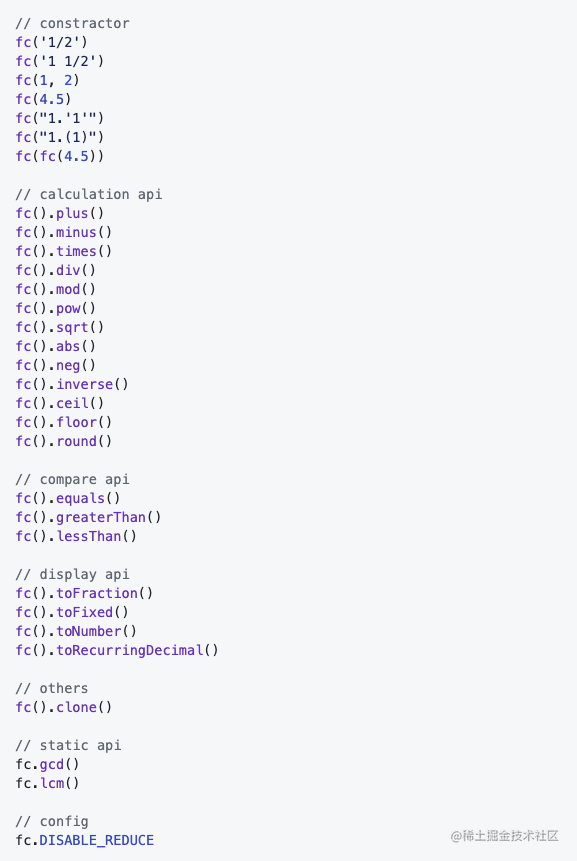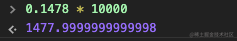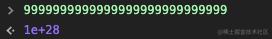# 学以致用：手把手教你撸一个工具库并打包发布，顺便解决JS小数计算不准问题

Math.sqrt(Math.pow(((1/3+3.5)*2/9-27/109)/(889/654),4));   //  0.1975308641975308


fc('1/3')
.plus(3.5)
.times('2/9')
.minus('27/109')
.div('889/654')
.pow(4)
.sqrt()
.toFraction();     // 输出： 16/81


fc('16/81').toRecurringDecimal(); // "0.(197530864)"


fc('0.(197530864)').toFraction(); // 16/81


0.1 + 0.2;     // 0.30000000000000004
fc(0.1).plus(0.2).toNumber(); // 0.3


npm install fraction-calculator --save


GitHub地址： github.com/dennis-jian…

GitHub上有详细的使用说明，本文接下来的篇幅会详细讲解怎么实现功能和打包发布。

## 功能实现

### API一览1. 构造器
2. 计算API
3. 比较API
4. 输出显示API
5. 静态API
6. 其他API
7. 配置

### 构造器

1. 不用new就可以直接使用
2. 支持字符串的分数，包括有整数部分或者没有整数部分
3. 支持数字
4. 支持循环小数
5. 支持另一个实例
6. 支持两个数字参数

#### 从去掉new开始构建架构

// 首先创建一个fc的函数，我们最终要返回的其实就是一个fc的实例
// 但是我们又不想让用户new，那么麻烦
// 所以我们要在构造函数里面给他new好这个实例，直接返回
function FractionCalculator(numStr, denominator) {
// 我们new的其实是fc.fn.init
return new FractionCalculator.fn.init(numStr, denominator);
}

// fc.fn其实就是fc的原型，算是个简写，所有实例都会拥有这上面的方法
FractionCalculator.fn = FractionCalculator.prototype = {};

// 这个其实才是真正的构造函数，这个构造函数也很简单，就是将传入的参数转化为分数
// 然后将转化的分数挂载到this上，这里的this其实就是返回的实例
FractionCalculator.fn.init = function(numStr, denominator) {
this.fraction = FractionCalculator.getFraction(numStr, denominator);
};

// 前面new的是init，其实返回的是init的实例
// 为了让返回的实例能够访问到fc的方法，将init的原型指向fc的原型
FractionCalculator.fn.init.prototype = FractionCalculator.fn;


// 添加实例方法这样写，下面是plus方法，注意这里是在fn上，也就是原型上
FractionCalculator.fn.plus = function() {}

// 添加静态方法这样写，下面是gcd方法，注意这里没在fn上
FractionCalculator.gcd = function() {}


let fraction = {
numerator,      // 分子
denominator,    // 分母
};


#### 支持浮点数，解决JS本身精度问题#### 循环小数转分数

5.45(689)

= 5 + 0.45 + 0.00(689)

= 5 + $\frac{45}{100}$ + (0.(689)/100)

= 5 + $\frac{45}{100}$ + ($\frac{689}{999}$/100)

= 5 + $\frac{45}{100}$ + $\frac{689}{99900}$

### 计算API

#### 链式调用

fc(1.5).plus('1/3').times(5).toNumber();

1. 前面讲了fc(1.5)返回的是一个fc的实例，为了能够让他调到plus，所以plus肯定得是一个实例方法
2. plus的返回值还能调到times方法，那plus的返回值到底是什么呢？答案还是fc实例，我们plus还得返回一个fc实例，times也是一个实例方法，所以plus的返回值能访问。
3. plus怎么返回一个fc实例呢？其实很简单，他自己就是实例方法，是被fc实例调用的，所以这个方法里面的this就指向了调用者，也就是fc实例。所以要实现链式调用，就要在对应的实例方法里面返回this。如果你对this指向还不是很熟悉，请看这篇文章。

function fc() {}

fc.prototype.func1 = function() { return this;}
fc.prototype.func2 = function() { return this;}

// 因为实例方法func1和func2都返回了this，所以可以一直点点点
const instance = new fc();
instance.func1().func2().func2().func1();


#### 约分和通分

$\frac{1}{2} + \frac{1}{3}$

= $\frac{3}{6} + \frac{2}{6}$

=$\frac{5}{6}$

gcd(a, b) = gcd(b, a %b )

gcd(150, 270)

= gcd(270, 150)

= gcd(150, 120)

= gcd(120, 30)

= gcd(30, 0)

function getGCD(a, b) {
// get greatest common divisor(GCD)
// GCD(a, b) = GCD(b, a % b)
a = Math.abs(a);
b = Math.abs(b);
let mod = a % b;

while (mod !== 0) {
a = b;
b = mod;
mod = a % b;
}

return b;
}


a = x * gcd

b = y * gcd

(x * gcd) * (y * gcd) / gcd

= a * b / gcd

#### 取余和取模

1. 先算商-1.75
2. 取余，商往0方向取整，也就是-1，然后算 -7 - (-1) * 4 = -3
3. 取模，商往负无穷方向取整，也就是-2， 然后算 -7 - (-2) * 4 = 1

JS的%其实是取余计算，所以fc的mod方法跟他保持了一致，是取余运算，算法跟前面的例子是一样的，计算过程中用到了我们前面实现的减法和乘法。

### 显示API

#### 输出成循环小数

$\frac{5}{3}$

= $\frac{15}{9}$

= $1 + \frac{6}{9}$

= 1.6666666666...

$\frac{3}{28}$ // 分母含质因子2，调整分子乘以10

-> $\frac{30}{28}$

= $\frac{15}{14}$ // 分母含质因子2，调整分子乘以10

-> $\frac{150}{14}$

= $\frac{75}{7}$

= $10 + \frac{5}{7}$

= $10 + \frac{714285}{999999}$

= 10.714285714285714285714285714285

-> 0.10(714285) // 前面乘了两个10，小数点左移两位function getDecimalsFromFraction(numerator, denominator) {
// make sure numerator is less than denominator
const modObj = {};
const quotientArray = [];

let mod;
let index = 0;

while (true) {
mod = numerator % denominator;

if (mod === 0) {
return quotientArray.join('');
}

let existIndex = modObj[mod];
if (existIndex >= 0) {
let quotientLength = quotientArray.length;
quotientArray.splice(existIndex, 0, '(');
quotientArray.splice(quotientLength + 1, 0, ')');

return quotientArray.join('');
}

modObj[mod] = index;
index++;
numerator = mod * 10;

let quotient = parseInt(numerator / denominator);
quotientArray.push(quotient);

if (index >= 3000) {
// Recurring part can be very long, we only handle first 3000 numbers
return quotientArray.join('');
}
}
}


### 静态API

fc有两个静态API，gcdlcm，这其实就是我们前面计算用到的最大公约数和最小公倍数，既然都写出来了，为啥不顺便暴露给用户用呢？

## 单元测试

describe('FractionCalculator instance', () => {
it('can support integer', () => {
const instance = fc(4);

expect(instance.fraction).toEqual({
numerator: 4,
denominator: 1,
});
});
});


## 打包发布

1. 流行的ES6，node.js要支持
2. 浏览器要支持
3. 老的浏览器，比如IE，尽量支持

{
...
library: 'fc',                 // 库名字，也是script引入时挂载到window的对象名字
libraryTarget: 'umd',          // 支持的引入方式，umd是包括ES6, node, 浏览器，AMD等
libraryExport: 'default',      // 默认导出的路径，我用export default导出的就写'default'
...
}


{
...
"useBuiltIns": "usage"     // 关键就是这个配置，这个只会添加用到了的polyfill
...
}


1. fraction-calculator.js没有压缩，没有polyfill的版本，供ES6和node使用，package.json里面的main也指向的这个包，这样用户npm安装之后，import或者require的就是这个文件
2. fraction-calculator.min.js压缩版的fraction-calculator.js，供高级浏览器使用，比如火狐，Chrome，高级浏览器自己支持ES6，就不用polyfill了，这个文件体积也最小，只有7kb
3. fraction-calculator.polyfill.min.js加了polyfill的fraction-calculator.min.js，体积会稍微大一点，供IE之类的使用。

## 总结

fc项目GitHub地址： github.com/dennis-jian…

“前端进阶知识”系列文章：juejin.cn/post/684490…

“前端进阶知识”系列文章源码GitHub地址： github.com/dennis-jian…